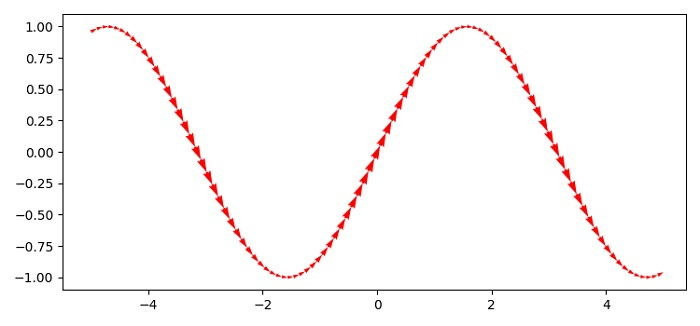# How do I specify an arrow-like linestyle in Matplotlib?

To draw an arrow-like linestyle in matplotlib, we can use quiver() method.

## Steps

• Set the figure size and adjust the padding between and around the subplots.

• Create x and y data points using numpy.

• Use quiver() method to draw a line.

• To display the figure, use show() method.

## Example

import numpy as np
import matplotlib.pyplot as plt

plt.rcParams["figure.figsize"] = [7.50, 3.50]
plt.rcParams["figure.autolayout"] = True

x = np.linspace(-5, 5, 100)
y = np.sin(x)

plt.quiver(x[:-1], y[:-1], x[1:]-x[:-1], y[1:]-y[:-1], scale_units='xy', angles='xy', scale=1, color='red')

plt.show()

## Output